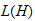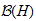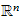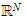# Publications

## Books in preparation

1. Apartness Spaces (with Luminiţa Vîţă), graduate monograph.

## Refereed/invited articles

There follows a list of my research papers, grouped under the following headings:

Algebra; Apartness and uniform spaces; Approximation theory; Constructive mathematics‒general articles; Constructive mathematics and physics; Constructive reverse mathematics; Dirichlet problem; Economic theory; Extended reviews; Functional analysis; Locally convex spaces; Measure and integration; Metric and normed spaces; Miscellaneous constructive papers; Real and complex analysis; Set theory

### Algebra

1. ‘On the derivation of xn in a ring’ (with Jeffrey Bergen), Proc. Amer. Math. Soc.90(1), 25–29, 1984.
2. ‘Prime and maximal ideals in constructive ring theory’, Comm. Algebra. 29(7), 2787–2803, 2001.
3. ‘The polydisk Nullstellensatz’ (with Ray Mines, Peter Schuster, and Fred Richman), Proc. Amer. Math. Soc. 132(7), 2133–2140, 2004.
4. ‘A simple constructive proof of Kronecker’s density theorem’ (with Peter Schuster), Elemente der Mathematik 61, 152–154, 2006.

### Apartness and uniform spaces

1. ‘Characterising near continuity constructively’ (with Luminiţa Vîţă), Math. Logic Quart. 27(4), 535–538, 2001.
2. ‘Apartness as a relation between subsets’ (with Peter Schuster and Luminiţa Vîţă), in: Combinatorics, Computability and Logic (Proceedings of DMTCS’01, Constanţa, Romania, 2–6 July 2001; C.S. Calude, M.J. Dinneen, S. Sburlan (eds.)), 203–214, DMTCS Series 17, Springer–Verlag, London, 2001.
3. ‘Cauchy nets in the constructive theory of apartness spaces’ (with Luminiţa Vîţă), Scientiae Mathematicae Japonicae 56, 123–132, 2002.
4. ‘Apartness, topology, and uniformity: a constructive view’ (with Peter Schuster and Luminiţa Vîţă), Computability and Complexity in Analysis (Proc. Dagstuhl Seminar 01461, 11–16 November 2001), Math. Log. Quart. 48, Suppl. 1, 16–28, 2002.
5. ‘Apartness spaces—a progress report’, European Assoc. for Theoretical Computer Science Bull. 81, 2003.
6. ‘Apartness spaces as a framework for constructive topology’ (with Luminiţa Vîţă), Ann. Pure Appl. Logic. 119(1–3), 61–83, 2003.
7. ‘Strong versus uniform continuity: a constructive round’ (with Peter Schuster and Luminiţa Vîţă), Quaest. Math. 26, 171–190, 2003.
8. ‘A constructive theory of point–set nearness’ (with Luminiţa Vîţă), Topology in Computer Science: Constructivity; Asymmetry and Partiality; Digitization (Proc. Dagstuhl Seminar 00231, 4-9 June 2000; R. Kopperman, M. Smyth, D. Spreen, eds.), Theoretical Computer Science 305 (1–3), 473–489, 2003.
9. ‘A proof–technique in uniform space theory’ (with Luminiţa Vîţă), 68(3), 795–802, 2003. (A corrigendum to this paper has been published in volume 69(1), p.328, 2004.)
10. ‘Cauchy nets in uniform apartness spaces’ (with Luminiţa Vîţă), Revue Romaine 48(5–6), 467–474, 2003.
11. ‘Strong and uniform continuity—the uniform space case’ (with Luminiţa Vîţă), London Math. Soc. J. Computing & Math.6, 326–334, 2003.
12. ‘Separatedness in constructive topology’ (with Luminiţa Vîţă), Documenta Mathematica 8, 567–576, 2003.
13. ‘More on Cauchy nets in apartness spaces’ (with Luminiţa Vîţă), Sci. Math. Japonicae 9, 375–380, 2004.
14. ‘A general constructive proof technique’ (with Luminiţa Vîţă), Elec. Notes in Theoretical Comp. Sci. 120, 31–43, 2005.
15. ‘Products in the category of apartness spaces’ (with Hajime Ishihara, Peter Schuster and Luminiţa Vîţă), Cahiers de Topologie et Géometrie Différentielle 46, 139–153, 2005.
16. ‘Strong continuity implies uniform sequential continuity’ (with Hajime Ishihara, Peter Schuster and Luminiţa Vîţă), Archive for Math. Logic 44(7), 887–895.
17. ‘A constructive treatment of Urysohn’s lemma in an apartness space’ (with Hannes Diener), Math. Logic Quarterly 52(5), 464–469, 2006.
18. ‘Pre-apartness structures on spaces of functions’ (with Luminiţa Vîţă), J. Complexity Theory (Special Issue, Proc. CCA 05 Kyoto)
19. ‘Almost locatedness in uniform spaces’ (with Hajime Ishihara, Ray Mines, Fred Richman, Peter Schuster, and Luminiţa Vîţă), Czech. Math. J.
20. ‘Journey into apartness space’ (with Luminiţa Vîţă), in: Logicism, Formalism, Intuitionism‒What has Become of Them? (S. Lindström, E. Palmgren, K. Segerberg, and V. Stoltenberg-Hansen, eds) Synthese Library, Springer–Verlag, Berlin, to appear in 2008.
21. ‘Product a–frames and proximity’, Math. Logic Quaterly 54(1), 12–25, 2008.
22. ‘Proximal connectedness’ (with Luminiţa Vîţă), Fundamenta Informaticae 83(1-2), 25–34, 2008.
23. ‘Apartness, compactness and nearness’ (with Hajime Ishihara, Peter Schuster, and Luminiţa Vîţă), Theoretical Comp. Sci. (special issue of proceedings of Dagstuhl 2006 topology meeting, to appear.

### Approximation theory

1. ‘On the foundations of best approximation theory’, J. Approx. Theory, 28(3), 273–292, 1980.
2. ‘A constructive development of Chebyshev approximation theory’, J. Approx. Theory 30(2), 99–120, 1981.
3. ‘A constructive analysis of the Remes algorithm’, J. Approx. Theory 32(4), 257–270, 1981.
4. ‘A constructive proximinality property of finite–dimensional linear spaces’, Rocky Mountain J. Math. 11(4), 491–497, 1981.
5. ‘Lipschitz constants and moduli of continuity for the Chebyshev projection’, Proc. Amer. Math. Soc. 85(4), 557–561, 1982.
6. ‘Recent progress in constructive approximation theory’, in The L.E.J. Brouwer Centenary Symposium (A.S. Troelstra and D. van Dalen, eds.), North–Holland, Amsterdam, 41–50, 1982.
7. ‘Approximate interpolation by functions in a Haar space’, J. Approx. Theory 57(1), 35–39, 1989.
8. ‘Constructing best approximations on a Jordan curve’ (with Wang Yuchuan), J. Approx. Theory 94(2), 222–234, 1998.
9. ‘Continuity and Lipschitz constants for projections’, J. Logic and Alg. programming, special issue for Dieter Spreen’s 60th birthday.

### Constructive mathematics‒general articles

1. ‘What is constructive mathematics?’ (with Ray Mines), Math. Intelligencer 6(4), 32–38, 1985.
2. Constructive Mathematics’, in Stanford Encyclopedia of Philosophy (ISSN 1095–5054).
3. ‘Paradise lost, or Paradise regained?’ (with Luminiţa Dediu), EATCS Bulletin 63, 141–152, October 1997.
4. ‘Constructive truth in practice’, in Truth in Mathematics (Proceedings of Conference held at Mussomeli, Sicily, 13–21 September 1995; H.G. Dales and G. Oliveri, eds), 53–69, Clarendon Press, Oxford, 1998.
5. ‘Constructive mathematics, in theory and programming practice’ (with Steve Reeves), Philosophia Mathematica 7(1), 65–104, 1999.
6. ‘Constructive mathematics: a foundation for computable analysis’, Theoretical Computer Science 219 (1–2), 95-109, 1999.
7. ‘Constructivity in mathematics’, Cubo (Math. Journal of Universidad de la Frontera, Chile) 6(1), 209–258, 2004.
8. ‘Errett Bishop’, in: From Sets and Types to Topology and Analysis (L. Crosilla and P. Schuster, eds), vii–ix, Oxford Logic Guides, Clarendon Press, Oxford, 2005.
9. ‘Church’s thesis and Bishop.s constructivism’, in: Church’s Thesis After 70 Years (Adam Olszewski, ed.), 58–65, Ontos Verlag, Berlin, 2006.
10. ‘Philosophy of mathematics: five questions’ in Philosophy of mathematics: five questions (V.F. Hendricks and H. Leitgeb, eds), 45–49, New York, London: Automatic Press/VIP, 2007.

### Constructive mathematics and physics

1. ‘Towards a constructive foundation for quantum mechanics’, in Constructive Mathematics (F. Richman, ed.), Springer Lecture Notes in Math. 873, 260–273, 1981.
2. ‘Constructive mathematics and unbounded operators—a reply to Hellman’, J. Philosophical Logic 24, 549–561, 1995.
3. ‘Can constructive mathematics be applied in physics?’, J. Philosophical Logic 28(5), 439-453, 1999.
4. ‘A constructive proof of Gleason’s theorem’ (Fred Richman and Douglas Bridges), Journal of Functional Analysis 162, 287-312, 1999.
5. ‘Constructive mathematics and quantum physics’ (with Karl Svozil), Int. J. Theor. Physics 39, 501-513 (2000).

### Constructive reverse mathematics

1. ‘The fan theorem and unique existence of maxima’ (with Josef Berger and Peter Schuster), J. Symbolic Logic 71(2), 713–720, 2006.
2. ‘A bizarre property equivalent to the Π–fan theorem’ (with Josef Berger), Logic Journal of IGPL, Advance Access published online, 19 August 2006.
3. ‘A fan–theoretic equivalent of the antithesis of Specker’s theorem’ (with Josef Berger), Proc. Koninklijke Nederlandse Akad. Wetenschappen (Indag. Math., N.S.), 18(20< 195–202, 2007.
4. ‘The pseudocompactness of [0, 1] is equivalent to the uniform continuity theorem’ (with Hannes Diener), J. Symbolic Logic 72(4), 1379–1383, 2008.
5. ‘The anti-Specker property, a Heine–Borel property, and uniform continuity’ (with Josef Berger), Archive for Math. Logic 46(7–8), 583–592, 2008.
6. ‘Continuity properties of preference relations’ (with Marian Baroni), Elec. Notes in Theor. Comp. Sci. 202, 19–25, 2008.
7. ‘Uniform continuity properties of preference relations’, Notre Dame Journal of Formal Logic 49(1), 97–106, 2008.
8. ‘A reverse look at Brouwer’s fan theorem’, Proc. Conference Cent Ans de I’Intuitionnisme (Cerisy, Normandy, June 2006).

### Dirichlet problem

1. ‘Constructing cutoff functions’ (with Wang Yuchuan), New Zealand J. Math. 26, 25–30, 1997.
2. ‘Constructive aspects of the Dirichlet problem’ (with Wang Yuchuan), J. Universal Comp. Sci. 3(11), 1148–1161, 1997.
3. ‘Weak solutions of the Dirichlet Problem and the Locatedness of’ (with Wang Yuchuan), New Zealand J. Math.28(1), 1–5, 1998.
4. ‘Constructive weak solutions of the Dirichlet Problem’ (with Wang Yuchuan), J. London Math. Soc. 57(3), 655–667 (Officially the June 1998 volume, but did not appear until January 1999).

### Economic theory

1. ‘Preference and utility—a constructive development’, J. Math. Economics 9, 165–185, 1982.
2. ‘A numerical representation of preferences with intransitive indifference’, J. Math. Economics 11, 25–42, 1983.
3. ‘Numerical representation of intransitive preferences on a countable set’, J. Economic Theory 30, 213–217, 1983.
4. ‘Representing interval orders by a single real–valued function’, J. Economic Theory 36(1), 149–155, 1985.
5. ‘Numerical representation of interval orders on a topological space’, J. Economic Theory 38(1), 160–166, 1986.
6. ‘The Euclidean distance construction of order homomorphisms’, Math. Social Sciences 15(2), 179–188, 1988.
7. ‘The constructive theory of preference orderings on a locally compact space’, Proc. Koninklijke Nederlandse Akad. Wetenschappen (Indag. Math.) 92 (2), 141–165, 1989.
8. ‘Preference, indifference, and Markov’s principle’, Math. Social Sciences 20, 131–146, 1990.
9. ‘Order isomorphisms: a constructive measure–theoretic view’, in Mathematical Logic: Proceedings of Heyting ’88 Conference in Logic (P.P. Petkov, ed.), 243–255, Plenum Publishing Corp., New York, 1990.
10. ‘The constructive inequivalence of various notions of preference ordering’, Math. Soc. Sciences 21, 169–176, 1991.
11. ‘A recursive counterexample to Debreu’s theorem on the existence of a utility function’ (with Fred Richman), Math. Soc. Sciences 21, 179–182, 1991.
12. ‘The construction of a continuous demand function for uniformly rotund preferences’, J. Math. Economics 21, 217–227, 1992.
13. ‘The constructive theory of preference orderings on a locally compact space II’, Math. Social Sciences 27, 1–9, 1994.
14. ‘Constructive methods in mathematical economics’, in Mathematical Utility Theory, J. Econ. (Zeitschrift für Nationalökonomie), Suppl. 8, 1–21, 1999.

### Extended reviews

The following extended reviews were commissioned.

1. A Course in Constructive Algebra (by R. Mines, F. Richman, and W. Ruitenburg), Bull. American Math. Soc. 20(1), 127–132, 1989.
2. Theories of Computational Complexity (by C.S. Calude), Kybernetes 19(3), 33–36, 1990.
3. Computability in Analysis and Physics (by M.B. Pour–El and J.A. Richards), Bull. Amer. Math. Soc. 24(1), 216–228, 1991.
4. Utility Maximization, Choice and Preference (F. Aleskerov, D. Bouyssou, and B. Monjardet), to appear in SIAM Review.

### Functional analysis

1. ‘On weak operator compactness of the unit ball of’, Zeit. math. Logik Grundlagen Math. 24, 493–494, 1978.
2. ‘A constructive look at positive linear functionals on’, Pacific J. Math. 95(1), 11–25, 1981.
3. ‘Operator ranges, integrable sets, and the functional calculus’, Houston J. Math. 11(1), 31–44, 1985.
4. ‘A constructive treatment of open and unopen mapping theorems’ (with W. Julian and R. Mines), Zeit. math. Logik Grundlagen Math. 35, 29–43, 1989.
5. ‘Locating the range of an operator on a Hilbert space’ (with Hajime Ishihara), Bull. London Math. Soc. 24, 599–605, 1992.
6. ‘Absolute continuity and the uniqueness of the constructive functional calculus’ (with Hajime Ishihara), Math. Logic Quarterly 40, 519–527, 1994.
7. ‘Spectra of selfadjoint operators in constructive analysis’ (with Hajime Ishihara), Proc. Koninklijke Nederlandse Akad. Wetenschappen (Indag. Math.) 7(1), 11–36, 1996.
8. ‘Constructing ultraweakly continuous linear functionals on’ (with Nicholas Dudley Ward), Proc. American Math. Soc. 126 (11), 3347–3353, 1998.
9. ‘A definitive constructive open mapping theorem?’ (with Hajime Ishihara), Math. Logic Quarterly 44, 545–552, 1998.
10. ‘Weak-operator continuity and the existence of adjoints’ (with Luminiţa Dediu), Math. Logic Quarterly 45(2), 203–206, 1999.
11. ‘The weak–operator sequential continuity of left multiplication’ (with Luminiţa Dediu), Proc. Koninklijke Nederlandse Akad. Wetenschappen (Indag. Math.).11(1), 39–42, 2000.
12. ‘A constructive analysis of a proof of that the numerical range is convex’ (with Robin Havea), London Math. Soc. Journal of Mathematics and Computation 3, 191–206, 2000.
13. ‘Constructive methods in Banach algebra theory’, Math. Japonica 52(1), 145–161, 2000.
14. ‘Adjoints, absolute values, and polar decompositions’ (with Fred Richman and Peter Schuster), J. Operator Theory 44, 243–254, 2000.
15. ‘Constructing extensions of ultraweakly continuous linear functionals’ (with Luminiţa Vîţă), J. Functional Analysis 178(2), 421–434, 2000.
16. ‘Constructive closed range and open mapping theorems’ (with Hajime Ishihara), Proc. Koninklijke Nederlandse Akad. Wetenschappen (Indag. Math.) 11(4), 509–516, 2001.
17. ‘A constructive version of the spectral mapping theorem’ (with Robin Havea), Math. Logic Quarterly 47(3), 299-304, 2001.
18. ‘Constructive unbounded operators’ (with Hajime Ishihara), in: Reuniting the Antipodes—Constructive and Nonstandard Views of the Continuum (Berger, Ulrich, Horst Osswald, and Peter Schuster, editors), 25–37, Proceedings of the Symposion in San Servolo/Venice, Italy, May 17-22, 1999, Synthèse Library, Kluwer Academic Publishers, Dordrecht, December 2001.
19. ‘Trace-class operators’ (with Fred Richman and Peter Schuster), Houston J. Math. 28, 565–583, 2002.
20. ‘Locating the range of an operator with an adjoint’ (with Hajime Ishihara and Bas Spitters), Proc. Koninklijke Nederlandse Akad. Wetenschappen (Indag. Math.) N.S., 11(3), 1–8, 2003.
21. ‘Approximating the numerical range in a Banach algebra’ (with Robin Havea), in: From Sets and Types to Topology and Analysis (L. Crosilla and P. Schuster, eds), 293–303, Oxford Logic Guides, Clarendon Press, Oxford, 2005.
22. ‘Constructive Banach algebra theory and the Nullstellensatz.’ (with Robin Havea and Peter Schuster), Publ. Math. Debrecen 69(1–2), 171–184, 2006.
23. ‘Ideals in constructive Banach algebra theory’ (with Robin Havea and Peter Schuster), J. Complexity 22, 729–737, 2006.
24. ‘Powers of a Hermitian element’ (with Robin Havea), New Zealand J. Math. 36, 1–10, 2007.

### Locally convex spaces

1. ‘Constructive notes on uniform and locally convex spaces’ (with Luminiţa Dediu), in Proceedings of 12th International Symposium, FCT’99 Iasi, Romania, Springer Lecture Notes in Computer Science 1684, 195–203, 1999.
2. ‘The constructive uniqueness of the locally convex topology on’ (with Luminiţa Vîţă), in: From Sets and Types to Topology and Analysis (L. Crosilla and P. Schuster, eds), 304–315, Oxford Logic Guides, Clarendon Press, Oxford, 2005.

### Measure and integration

1. ‘The constructive Radon–Nikodým theorem’, Pacific J. Math. 70(1), 51–65, 1977.
2. ‘On the constructive convergence of series of independent functions’, Zeit. math. Logik Grundlagen Math. 25, 93–96, 1979.
3. ‘Locatedness, convexity, and Lebesgue measurability’, Quart. J. Math. Oxford (2) 39, 411–421, 1988.
4. ‘On the Lebesgue measurability of continuous functions in constructive analysis’ (with Osvald Demuth), Bull. Amer. Math. Soc. 24(2), 259–276, 1991.
5. ‘Colocatedness and Lebesgue interability’ in: Computation and Logic in the Real World (proc. 3rd Conference on Computability in Europe, Siena, 2007; S.B. Cooper, L. Löwe, A. Sorbi, eds), Lecture Notes in Computer Science 4497, 98–104, Springer–Verlag, Berlin–Heidelberg, 2007.

### Metric and normed spaces

1. ‘Some notes on continuity in constructive analysis’, Bull. London Math. Soc. 8, 179–182, 1976.
2. ‘A constructive look at orthonormal bases in Hilbert space’, Amer. Math. Monthly, 84(3), 189–191, 1977.
3. ‘On the connectivity of convex sets’, Bull. London Math. Soc. 10, 86–90, 1978.
4. ‘More on the connectivity of convex sets’, Proc. Amer. Math. Soc. 68(2), 214–216, 1978.
5. ‘On continuous mappings between locally compact metric spaces’, Bull. London Math. Soc. 10, 201–208, 1978.
6. ‘A criterion for compactness in metric spaces?’, Zeit. math. Logik Grundlagen Math. 25, 97–98, 1979.
7. ‘Connectivity properties of metric spaces’, Pacific J. Math. 80(2), 325–333, 1979.
8. ‘Compactly generated Banach spaces’ (with F. Richman, A. Calder, W. Julian, and R. Mines), Arch. Math. 36, 239–243, 1981.
9. ‘Locating metric complements in’ (with A. Calder, W. Julian, R. Mines, and F. Richman), in Constructive Mathematics (F. Richman, ed.), Springer Lecture Notes in Math. 873, 241–249, 1981.
10. ‘Bounded linear mappings of finite rank’ (with A. Calder, W. Julian, R. Mines, and F. Richman), J. Functional Anal. 43(2), 143–148, 1981.
11. ‘Linear mappings are fairly well–behaved’ (with Hajime Ishihara), Arch. Math. 54, 558–562, 1990.
12. ‘Extensions and fixed points of contractive mappings in’ (with F. Richman, W. Julian, and R. Mines), J. Math. Analysis and Applications 165(2), 438–456, 1992.
13. ‘Sequential, pointwise, and uniform continuity: a constructive note’, Math. Logic Quarterly 39, 55–61, 1993.
14. ‘Constructive notions of strict convexity’, Math. Logic Quarterly 39, 295–300, 1993.
15. ‘Complements of intersections in constructive mathematics’ (with Hajime Ishihara), Math. Logic Quarterly 40, 35–43, 1994.
16. ‘Sets, complements and boundaries’ (with Fred Richman and Wang Yuchuan), Proc. Koninklijke Nederlandse Akad. Wetenschappen (Indag. Math.) 7(4), 425–445, 1996.
17. ‘Sequentially continuous linear mappings in constructive analysis’ (with Ray Mines), J. Symbolic Logic 63(2), 579–583, 1998.
18. ‘Weak continuity properties in constructive analysis’ (with Luminiţa Dediu), Logic Journal of the IGPL 7(3), 277–281, 1999.
19. ‘Sequential Compactness in Constructive Analysis’ (with Hajime Ishihara and Peter Schuster), Österr. Akad. Wiss. Math.–Natur. Kl. Sitzungsber. II. 208, 159–163, 1999 (published in July 2000).
20. ‘Sequential continuity of functions in recursive analysis’ (with Ayan Mahalanobis), Mathematical Logic Quarterly 46(1), 2000, 139-143.
21. ‘Converses of Bishop’s lemma on located sets’, Proc. Koninklijke Nederlandse Akad. Wetenschappen (Indag. Math.). 11(1), 31–38, 2000.
22. ‘Increasing, nondecreasing, and virtually continuous functions’ (with Ayan Mahalanobis), J Automata, Languages and Combinatorics 6(2), 139–143, 2001.
23. ‘Dini’s Theorem: a constructive case study’, in: Combinatorics, Computability and Logic (Proceedings of DMTCS’01, Constanţa, Romania, 2–6 July 2001; C.S. Calude, M.J. Dinneen, S. Sburlan (eds.)), 69–80, DMTCS Series 17, Springer–Verlag, London, 2001.
24. ‘Compactness and continuity, constructively revisited’ (with Hajime Ishihara and Peter Schuster), in: Computer Science Logic (J. Bradfield, ed; Proceedings of 16th International Workshop CSL 2002, 11th Annual Conference of the EACSL, Edinburgh, Scotland, September 22–25), Lecture Notes in Computer Science 2471, 89–102, Springer–Verlag, Berlin and Heidelberg, 2002.
25. ‘Kernels of seminorms in constructive analysis’ (with Nicholas F. Dudley Ward), Theoretical Comp. Sci. 284, 259–267, 2002.
26. ‘Exact, continuous boundary crossings out of convex sets in’ (with Gabriela Popa), Quart. J. Math. 54, 391–398, 2003.
27. ‘Ishihara’s proof technique in constructive analysis’ (with Dirk van Dalen and Hajime Ishihara), Proc. Koninklijke Nederlandse Akad. Wetenschappen (Indag. Math.) N.S., 14(2), 163–168, 2003.
28. ‘Constructive complements of unions of two closed sets’, Math. Logic Quarterly 50(3), 293–294, 2004.
29. ‘Computing infima on convex sets, with applications in Hilbert space’ (with Hajime Ishihara and Luminiţa Vîţă), Proc. Amer. Math. Soc. 132(9), 2723–2732, 2004.
30. ‘A new constructive version of Baire’s Theorem’ (with Hajime Ishihara and Luminiţa Vîţă), Hokkaido Math. Journal 35(1), 107–118, 2006.

### Miscellaneous constructive papers

1. ‘On recursive bounds for the exceptional values in speed–up’ (with Cristian Calude), Theoretical Computer Science 132, 387–394, 1994.
2. ‘Linear independence without choice’ (with Fred Richman and Peter Schuster), Annals of Pure and Applied Logic 101(1), 95–102, 1999.
3. ‘A weak countable choice principle’ (with Fred Richman and Peter Schuster), Proc. Amer. Math. Soc. 128(9), 2749–2752, 2000.
4. ‘First steps in constructive game theory’, Math. Logic Quarterly 50(4/5), 501–506, 2004.
5. ‘Reality and virtual reality in Mathematics’, in: Millennium III (C.S. Calude and K. Svozil, eds), Black Sea University and Romanian Academy, Bucharest, Romania, Autumn 2001. Also in Bull. European Assoc. for Theoretical Computer Science 78, 221–230, 2002.

### Real and complex analysis

1. ‘Geometric intuition and elementary constructive analysis’, Zeit. math. Logik Grundlagen Math. 25, 521–523, 1979.
2. ‘On Montel’s proof of the Great Picard theorem’, Amer. Math. Monthly 88(1), 45–47, 1981.
3. ‘On the isolation of zeroes of an analytic function’, Pacific J. Math. 96(1), 13–22, 1981.
4. ‘Picard’s theorem’ (with A. Calder, W. Julian, R. Mines, and F. Richman), Trans. Amer. Math. Soc. 269(2), 513–520, 1982.
5. ‘A general constructive intermediate value theorem’, Zeit. math. Logik Grundlagen Math. 35, 433–435, 1989.
6. ‘A constructive look at the real number line’, in Synthèse: Real Numbers, Generalizations of the Reals and Theories of Continua (P. Ehrlich, ed.), pp. 29–92, Kluwer Academic Publishers, Amsterdam, 1994.
7. ‘The constructive implicit function theorem and applications in physics’ (with C. Calude, B. Pavlov, and D. Ştefănescu), Chaos, Solitons & Fractals 10(6), 927–934, 1999.
8. ‘A constructive look at functions of bounded variation’, Bull. London Math. Soc. 32(3), 316–324, 2000.
9. ‘Bounded variation implies regulated: a constructive proof’ (with Ayan Mahalanobis), J. Symbolic Logic.66(4), 1695–1700, 2001.
10. ‘Constructing local optima on a compact interval’, to appear in Archiv für Math. Logik.

### Set theory

1. ‘A note on Morse’s lambda notation in set theory’, Zeit. math. Logik Grundlagen Math. 24, 113–114, 1978.
2. ‘A constructive Morse theory of sets’, in Mathematical Logic and its Applications (D. Skordev, ed.), Plenum Publishing Corp., New York, 61–79, 1987.